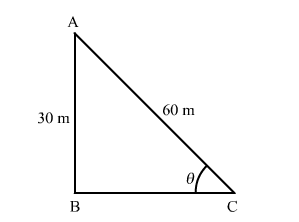# Choose the correct answer of the following question:`
Question:

Choose the correct answer of the following question:

A kite is flying at a height of 30 m from the ground. The length of string from the kite to the ground is 60 m. Assuming that there is no slack
in the string, the angle of elevation of the kite at the ground is

(a) 45°                   (b) 30°                   (c) 60°                   (d) 90°

Solution:Let point A be the position of the kite and AC be its string.

We have,

$\mathrm{AB}=30 \mathrm{~m}$ and $\mathrm{AC}=60 \mathrm{~m}$

Let $\angle \mathrm{ACB}=\theta$

In $\triangle \mathrm{ABC}$,

$\sin \theta=\frac{\mathrm{AB}}{\mathrm{AC}}$

$\Rightarrow \sin \theta=\frac{30}{60}$

$\Rightarrow \sin \theta=\frac{1}{2}$

$\Rightarrow \sin \theta=\sin 30^{\circ}$

$\therefore \theta=30^{\circ}$

Hence, the correct answer is option (b).